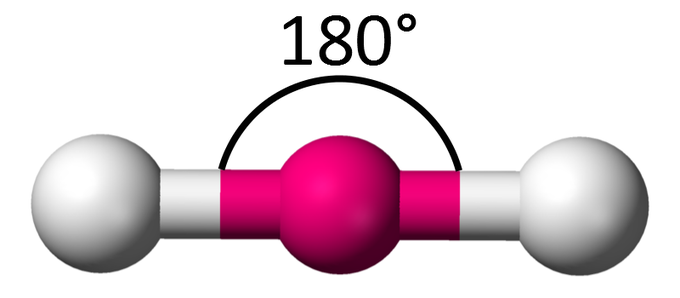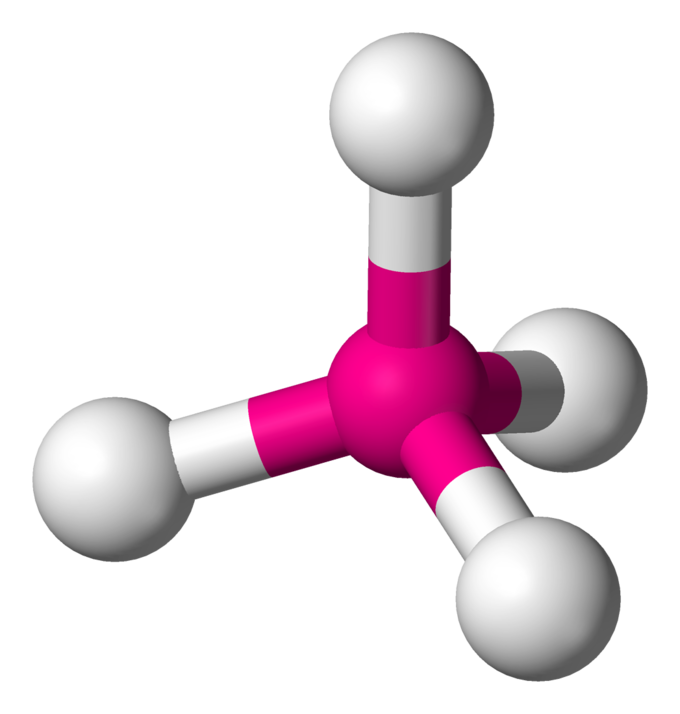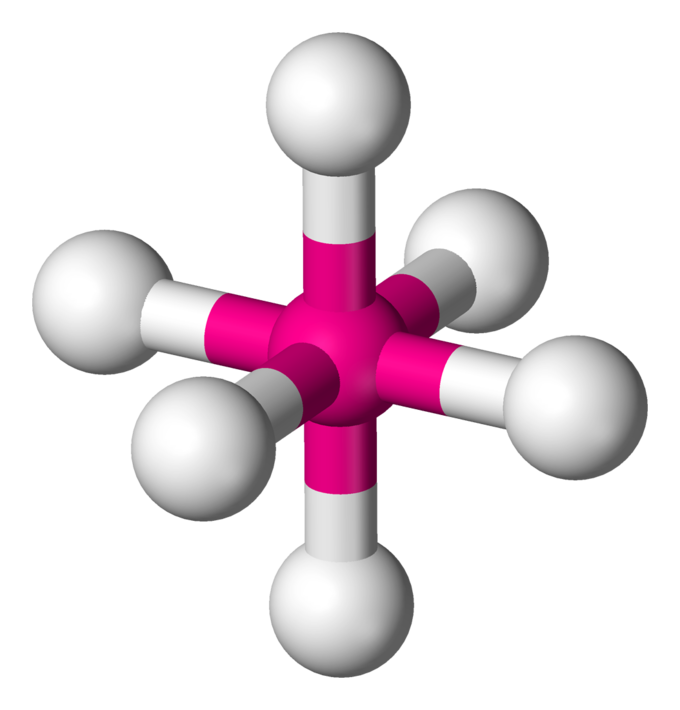## Applying the VSEPR Model

#### Learning Objective

• Apply the VSEPR model to determine a molecule’s geometry.

#### Key Points

• Electron repulsion between orbitals dictates molecular geometry.
• Nonbonding electron orbitals differ from bonding orbitals because nonbonding orbitals are concentrated closer to the central atom.
• Nonbonding orbitals exert more repulsion on other orbitals; for example, although the oxygen is a tetrahedral in H2O, the molecule’s shape is bent.

#### Terms

• coordination numberthe total number of atoms that directly neighbor a central atom in a molecule or ion
• repulsiona force that moves two bodies away from each other

## VSEPR

The valence shell electron pair repulsion (VSEPR) model predicts the shape of individual molecules based on the extent of electron-pair electrostatic repulsion. According to VSEPR, the valence electron pairs surrounding an atom mutually repel each other; they adopt an arrangement that minimizes this repulsion, thus determining the molecular geometry. This means that the bonding (and non-bonding) electrons will repel each other as far away as geometrically possible. The number of atoms bonded to a central atom combined with the number of pairs of its nonbonding valence electrons is called its steric number.

## Nonbonding Electrons and the Coordination Number

When calculating the coordination number around the central atom, count both the bonded atoms and the nonbonding pairs, because the nonbonding electrons are also in orbitals that occupy space and repel the other orbitals. For example, a water molecule (H2O) is not a linear molecule, because the Lewis electron dot formula predicts that there will be two pairs of nonbonding electrons.Water’s Lewis dot structureNotice the two lone pairs of electrons on the oxygen atom.

The oxygen atom will therefore be tetrahedrally coordinated, meaning that it sits at the center of a tetrahedron. Two of the coordination positions are occupied by the shared electron-pairs that constitute the O–H bonds, and the other two by the non-bonding pairs. Therefore, although the oxygen atom is tetrahedrally coordinated, the bonding geometry (shape) of the H2O molecule is described as bent.

There is an important difference between bonding and non-bonding electron orbitals. Because a nonbonding orbital has no atomic nucleus at its far end to draw the electron cloud toward it, the charge will be concentrated closer to the central atom; therefore, nonbonding orbitals exert more repulsion on other orbitals than do bonding orbitals.

In H2O for example, the two nonbonding orbitals push the bonding orbitals closer together, making the H–O–H angle 104.5° instead of the tetrahedral angle of 109.5°. Although the water molecule is electrically neutral, it is not electrically uniform; the non-bonding electrons create a higher concentration of negative charge at the oxygen end, making the hydrogen side relatively positive. This unbalanced charge is the root of many of water’s so-called anomalous properties.

## Linear Molecules

A triatomic molecule of the type AX2 has its two bonding orbitals 180° apart, producing a molecule of linear geometry; examples of this include BeCl2 and CO2. In the electron dot formula for carbon dioxide, the C-O bonds are double bonds. This makes no difference to VSEPR theory; the central carbon atom is still joined to two other atoms, and the electron clouds that connect the two oxygen atoms are 180° apart.Linear moleculeA simple triatomic molecule of the type AX2 has its two bonding orbitals 180° apart, producing a molecule of linear geometry.

## Trigonal Molecules

In an AX3 molecule, such as BF3, three regions of electron density extend out from the central atom. The repulsion between these will be at a minimum when the angle between any two is 120°. This requires that all four atoms be in the same plane; the resulting shape is called trigonal planar, or simply trigonal.The Lewis dot structure for boron trifluorideThe bond angles between the fluorine atoms are all 120°.

## Tetrahedrally-Coordinated Carbon Chains

In an AX4 molecule such as methane (CH4), a central atom is bonded to four other atoms. The four equivalent bonds point in four geometrically equivalent directions in three dimensions corresponding to the four corners of a tetrahedron; this is called tetrahedral coordination. The angle between any two bonds will be 109.5°. The bonding geometry will not be tetrahedral when the valence shell of the central atom contains nonbonding electrons.Tetrahedral moleculeIn a tetrahedral molecule, four equivalent bonds point in four geometrically equivalent directions in three dimensions, corresponding to the four corners of a tetrahedron centered on the carbon atom. Each bond angle measures 109.5 °C.

## Central Atoms with Five Bonds

Compounds of the type AX5 are formed by some of the elements in Group 15 of the periodic table. Examples of these compounds include PCl5 and AsF5.

Molecules with a coordination number of 5 are in the shape of a trigonal bipyramid; this consists of two triangular-based pyramids joined base-to-base. For example, in a PCl5 molecule, three of the chlorine atoms are in the central phosphorus atom’s plane (equatorial positions), while the other two atoms are above and below this plane (axial positions).The Lewis dot structure for phosphorous pentachlorideThe equatorial atoms have bond angles of 120°. The axial atoms are 180° away from each other. The axial atoms are 90° away from the equatorial atoms.

Equatorial and axial atoms have different geometrical relationships to their neighbors, and thus differ slightly in their chemical behavior. In 5-coordinated molecules containing lone pairs, these non-bonding orbitals will preferentially reside in the equatorial plane at 90° angles, with respect to no more than two axially-oriented bonding orbitals. An AX4E molecule (one in which the central atom is coordinated to four other atoms and to one nonbonding electron pair) has a “see-saw” shape; substituting more nonbonding pairs for bonded atoms reduces the triangular bipyramid coordination to even simpler molecular shapes.

## Octahedral Coordination

In an AX6 molecule, six electron pairs will try to point toward the corners of an octahedron (two square-based pyramids joined base-to-base). The shaded plane shown in the figure is only one of three equivalent planes defined by a four-fold symmetry axis. All the ligands are geometrically equivalent with bond angles of 90°; there are no separate axial and equatorial positions.

A coordination number of 6 is one of the most commonly encountered in inorganic chemistry, specifically in transition metal hydrates such as Fe(H2O)63+. There are well known examples of 6-coordinate central atoms with one, two, and three lone pairs.Octahedral moleculeIn an octahedral molecule, six electron pairs will try to point toward the corners of an octahedron.VSEPR tableUse the molecule’s formula, along with the number of bonded atoms and lone pair electrons, to determine its geometry.